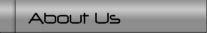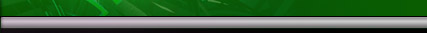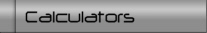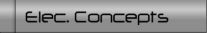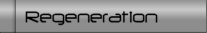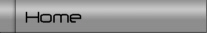### .: calculators useful for electric vehicle conversions

Calculate the effect of tire sizes, gear ratio, and battery pack requirements

# .: adding regeneration to a dc system

How to add braking regeneration to a car with a DC motor.

• Requirements for using a high output generator
• The problems and effect of a DC motor with advanced brush timing

# .: batteries

Making sense of battery ratings and how to use that information

# .: electrical concepts

• Current capacity for busbar and cables
• Diodes
• Fuses & fusing
• Power (watts)
• Resistors
• Series and parallel circuits
• Transformers (dc-dc converter)

 Some Useful Equations V  = I  x R (volts = amps x resistance) P  = I  x V (power (watts) = amps x volts) 746 watts  = 1 Horsepower Horsepower  =(RPM  x Lbs-Ft ) / 5252 (mechanical Hp) Newton-meter(Nm) = 0.73756 lb-ft example: 100 Nm * 0.73756 = 73.756 lb-ft Kilometers(km) = 0.6215 miles example: 10km * 0.6215 = 6.215 miles